# QUADRATIC EQUATION - Concept, Properties with Example

INTRODUCTION

The algebraic expression of the form ax2 + bx + c, a 0 is called a quadratic expression, because the highest order term in it is of second degree. Quadratic equation means, ax2 + bx + c = 0. In general whenever one says zeroes of the expression ax2+bx + c, it implies roots of the equation ax2 + bx + c = 0, unless specified otherwise.

A quadratic equation has exactly two roots which may be real (equal or unequal) or imaginary.

SOLUTION OF QUADRATIC EQUATION & RELATION BETWEEN ROOTS & CO-EFFICIENTS

• The general form of quadratic equation is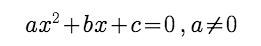The roots can be found in following manner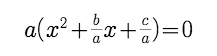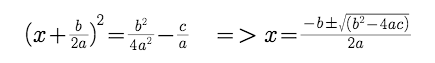This expression can be directly used to find the two roots of a quadratic equation.

• The expression b2 – 4 ac = D is called the discriminant of the quadratic equation
• if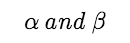are the roots of the quadratic equation then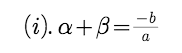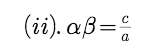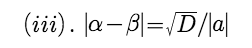•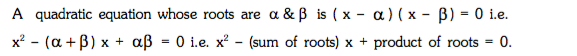Example :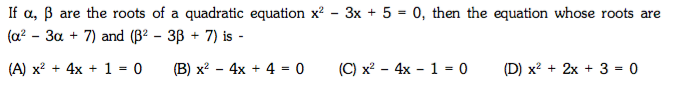Solution :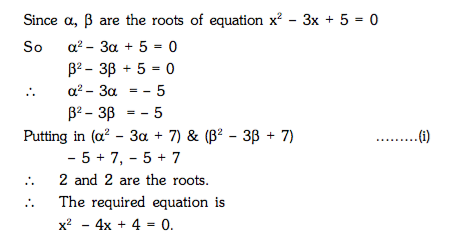Nature of Roots :

(a). Consider the quadratic equation ax2 + bx + c = 0 where a,b,c are real number a!=0 then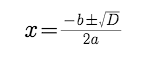1. D > 0 => roots are real & distinct (unequal).
2. D = 0 => roots are real & coincident (equal).
3. D < 0 => roots are imaginary.
4. if p + i q is one root of a quadratic equation, then the other root must be the conjugate p–iq&vice versa.

(b).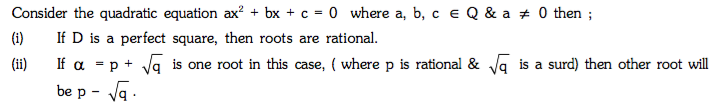Example :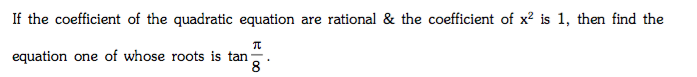Solution :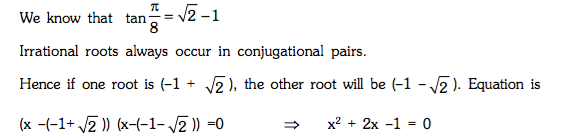Example :

Find all the integral values of a for which the quadratic equation (x – a)(x – 10) + 1 = 0 has integral roots.

Solution :

Here the equation is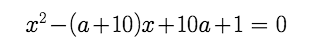Since integral roots will always be rational it means D should be a perfect square.

So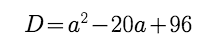If D is a perfect square it means we want difference of two perfect square as 4 which is possible only when

(a–10)(a-10) = 4 and D = 0.

so

(a-10) = +2 or (a-10) = -2

value of a should be 12, 8

ROOTS UNDER PARTICULAR CASES